### 4Position and Direction Vectors

A vector in 3D space is uniquely identified by three real numbers called coordinates or components. For example, the coordinates of (pos 1 2 3) are 1, 2 and 3.

Pict3D distinguishes between two kinds of vectors:
• A position vector, which is a value of type Pos, represents an absolute position in 3D space.

• A direction vector, which is a value of type Dir, represents a direction, with distance, in 3D space.

Generally, think of direction vectors as how to get from one position vector in space to another.

Suppose we define a direction vector dv1 as
 > (define dv1 (dir 1 1 0))
To picture it, we need a starting position vector. We’ll arbitrarily choose the origin, which is (pos 0 0 0).
 > (define v origin) > (arrow v dv1)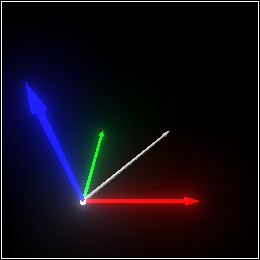We can use the direction vector dv1 to move the center of a sphere.
 > (define move-once (combine (arrow v dv1) (sphere v 0.1) (sphere (pos+ v dv1) 0.1)))
> move-once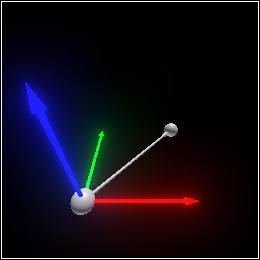We might even define another direction vector dv2 (pointing upward) to move it again.
> (define dv2 (dir 0 0 1))
 > (define move-twice (combine move-once (arrow (pos+ v dv1) dv2) (sphere (pos+ (pos+ v dv1) dv2) 0.1)))
> move-twiceBut we don’t have to move it twice: we can move it just once, by adding the direction vectors dv1 and dv2.
> (define dv (dir+ dv1 dv2))
 > (define move-twice-by-moving-once (combine (arrow v dv) (sphere v 0.1) (sphere (pos+ v dv) 0.1)))
 > (combine move-twice-by-moving-once (set-color move-twice (rgba "red" 0.75)))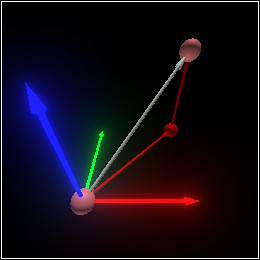Composing transformations—not just movement, but all parallel-line-preserving transformations—is discussed in the section Transformation.

Directions are used to move entire scenes, not just points:
 > (move (combine (with-color (rgba "red" 0.5) (cube origin 1/3)) (sphere origin 0.05)) (dir 1/2 1/2 0))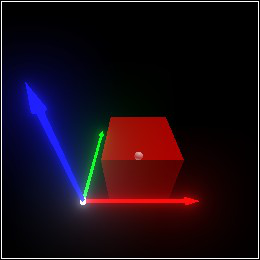When used as scaling factors, as in (rectangle origin (dir 1/3 1/3 1)), directions represent moving the center to a corner:
 > (move (combine (with-color (rgba "red" 0.5) (rectangle origin (dir 1/3 1/3 1))) (sphere origin 0.05) (arrow origin (dir 1/3 1/3 1))) (dir 1/2 1/2 0))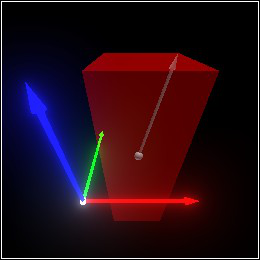#### 4.1Direction Vectors

 type
 predicate
The type and predicate of direction vectors.

 procedure(dir v) → Dir v : (U FlVector (Listof Real) (Vectorof Real)) (dir dx dy dz) → Dir dx : Real dy : Real dz : Real
Constructs a direction vector from its components dx, dy and dz, or converts a vector v in another representation to a direction vector.

 valuezero-dir : Dir = (dir 0 0 0)
The zero direction. Analogous to origin.

 value+x : Dir = (dir  1  0  0)
 value-x : Dir = (dir -1  0  0)
 value+y : Dir = (dir  0  1  0)
 value-y : Dir = (dir  0 -1  0)
 value+z : Dir = (dir  0  0  1)
 value-z : Dir = (dir  0  0 -1)
The positive and negative coordinate axis directions.

Example:
 > (with-color (rgba "black") (combine (with-emitted (emitted "cyan" 2) (arrow origin -x)) (with-emitted (emitted "magenta" 2) (arrow origin -y)) (with-emitted (emitted "yellow" 2) (arrow origin -z))))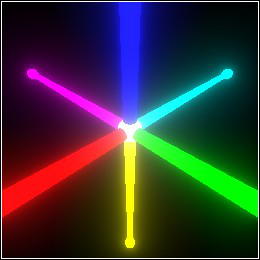Alternatively, these are the face normals for an axis-aligned rectangle, or the directions from the center of a (cube v 1) to the centers of each of its faces.

 value+x+y : Dir = (dir  1  1  0)
 value+x-y : Dir = (dir  1 -1  0)
 value+y+z : Dir = (dir  0  1  1)
 value+y-z : Dir = (dir  0  1 -1)
 value+x+z : Dir = (dir  1  0  1)
 value+x-z : Dir = (dir  1  0 -1)
 value-x+y : Dir = (dir -1  1  0)
 value-x-y : Dir = (dir -1 -1  0)
 value-y+z : Dir = (dir  0 -1  1)
 value-y-z : Dir = (dir  0 -1 -1)
 value-x+z : Dir = (dir -1  0  1)
 value-x-z : Dir = (dir -1  0 -1)
The directions from the center of a (cube v 1) to the centers of each of its edges.
 > (combine (cube origin 1 #:inside? #t) (with-color (rgba "cyan") (for/list ([dv  (list +x+y +x-y +y+z +y-z +x+z +x-z -x+y -x-y -y+z -y-z -x+z -x-z)]) (arrow origin dv))) (basis 'camera (point-at (pos 2 0.5 0.75) origin)))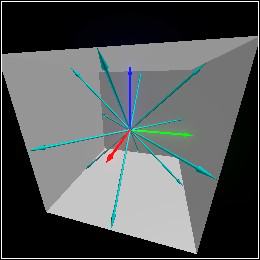value+x+y+z : Dir = (dir  1  1  1)
 value+x+y-z : Dir = (dir  1  1 -1)
 value+x-y+z : Dir = (dir  1 -1  1)
 value+x-y-z : Dir = (dir  1 -1 -1)
 value-x+y+z : Dir = (dir -1  1  1)
 value-x+y-z : Dir = (dir -1  1 -1)
 value-x-y+z : Dir = (dir -1 -1  1)
 value-x-y-z : Dir = (dir -1 -1 -1)
The directions from the center of a (cube v 1) to each of its corners.
 > (combine (cube origin 1 #:inside? #t) (with-color (rgba "magenta") (for/list ([dv  (list +x+y+z +x+y-z +x-y+z +x-y-z -x+y+z -x+y-z -x-y+z -x-y-z)]) (arrow origin dv))) (basis 'camera (point-at (pos 2 0.5 0.75) origin)))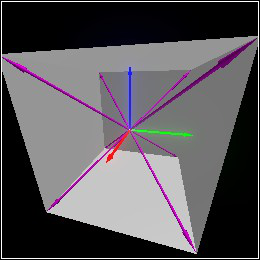procedure(dir+ dv1 dv2) → Dir dv1 : Dir dv2 : Dir
 procedure(dir-scale dv s) → Dir dv : Dir s : Real
 procedure(dir-negate dv) → Dir dv : Dir
 procedure(dir- dv1 dv2) → Dir dv1 : Dir dv2 : Dir
(dir+ dv1 dv2) composes movement in the directions dv1 and dv2 by adding their components.

(dir-scale dv s) scales the direction dv by multiplying each component by s.

(dir-negate dv) returns the direction opposite dv by negating each component, and is equivalent to (dir-scale dv -1).

(dir- dv1 dv2) is equivalent to (dir+ dv1 (dir-negate dv2)).

 procedure(dir-dist dv) → Flonum dv : Dir
 procedure(dir-dist^2 dv) → Flonum dv : Dir
Return the distance and squared distance represented by dv.

 procedure(dir-norm dv p) → Flonum dv : Dir p : Nonnegative-Real
Return the distance represented by dv under the L-p norm.

 procedure(dir-normalize dv) → (U #f Dir) dv : Dir
Returns a new direction vector in the same direction as dv, but distance 1. If dv is (dir 0 0 0), (dir-normalize dv) returns #f.

 procedure(dir-dot dv1 dv2) → Flonum dv1 : Dir dv2 : Dir
Returns the dot product of dv1 and dv2.

 procedure(dir-cross dv1 dv2) → Dir dv1 : Dir dv2 : Dir
Returns the cross product of dv1 and dv2.

 procedure(dir-project dv1 dv2) → (U #f Dir) dv1 : Dir dv2 : Dir
Returns the projection of dv1 onto dv2, or #f if dv2 is (dir 0 0 0).

 procedure(dir-reject dv1 dv2 [s]) → Dir dv1 : Dir dv2 : Dir s : Real = 1
Returns the rejection of dv1 from dv2. Equivalent to

(dir- dv1 (dir-scale (dir-project dv1 dv2) s))

when (dir-project dv1 dv2) doesn’t return #f.

 procedure(dir-reflect dv1 dv2) → Dir dv1 : Dir dv2 : Dir
Reflects dv1 in the direction dv2. Equivalent to (dir-reject dv1 dv2 2).

If dv is the velocity of an object that hits an immovable surface with normal n, then (dir-reject dv n) is the object’s new velocity, assuming an ideal elastic collision.

In other words, use this function to bounce things off walls in games.

 procedure(angles->dir yaw pitch) → Dir yaw : Real pitch : Real
Converts yaw (aka longitude, or rotation about the z axis) and pitch (aka latitude, or rotation about the x axis) into a direction with distance 1. Both angles are measured in degrees. Yaw is applied first.

 procedure dv : Dir
Converts a direction dv into yaw (aka longitude) and pitch (aka latitude). This is the inverse of angles->dir.

 procedure(dir-dx dv) → Flonum dv : Dir
 procedure(dir-dy dv) → Flonum dv : Dir
 procedure(dir-dz dv) → Flonum dv : Dir
Return the components of dv.

You’ll usually want to use match or match-define instead:
 > (match-define (dir dx dy dz) (dir 1 2 3)) > (list dx dy dz) '(1.0 2.0 3.0)

#### 4.2Position Vectors

 type
 predicate
The type and predicate of position vectors.

 procedure(pos v) → Pos v : (U FlVector (Listof Real) (Vectorof Real)) (pos x y z) → Pos x : Real y : Real z : Real
Constructs a position vector from its coordinates x, y and z, or converts a vector v in another representation to a position vector.

 valueorigin : Pos = (pos 0 0 0)
The origin. If 3D space has a center, this is it. Analogous to zero-dir.

 procedure(pos+ v dv [s]) → Pos v : Pos dv : Dir s : Real = 1.0
Moves v in direction dv optionally scaled by s. (pos+ v dv s) is equivalent to (pos+ v (dir-scale dv s)), but more accurate when one of the resulting components is near 0.0.

 procedure(pos- end start) → Dir end : Pos start : Pos
Returns the direction to move in to get from start to end; i.e. the dv for which (pos+ start dv) is end.

 procedure(pos-between start end t) → Pos start : Pos end : Pos t : Real
Interpolates between start and end by fraction t. The fraction t should be between 0 and 1, but does not have to be.

Example:
 > (combine (with-color (rgba "red") (sphere (pos 1 0 0) 1/10)) (with-color (rgba "blue") (sphere (pos 0 1 1) 1/10)) (for/list ([t  (in-range 1/10 1 1/10)]) (define v (pos-between (pos 1 0 0) (pos 0 1 1) t)) (sphere v 1/20)))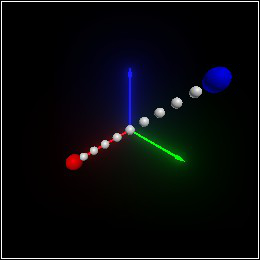procedure(pos-dist v1 v2) → Flonum v1 : Pos v2 : Pos
 procedure(pos-dist^2 v1 v2) → Flonum v1 : Pos v2 : Pos
Return the distance and squared distance between v1 and v2.

 procedure(pos-x v) → Flonum v : Pos
 procedure(pos-y v) → Flonum v : Pos
 procedure(pos-z v) → Flonum v : Pos
Return the coordinates of v.

You’ll usually want to use match or match-define instead:
 > (match-define (pos x y z) (pos 1 2 3)) > (list x y z) '(1.0 2.0 3.0)